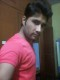Arun ChandravanshiAndroid RecyclerView OnItemClickListener Example

If you’ve used a RecyclerView, you may know that they don’t have a setOnItemClickListener as ListView had, so we have to create our own way to do it. There are many ways to achieve this, but I will show you the way I usually do it. My mechanism consists of passing the listener as a parameter to the constructor …

Android RecyclerView Example

RecyclerView The RecyclerView widget is a more advanced and flexible version of ListView. This widget is a container for displaying large data sets that can be scrolled very efficiently by maintaining a limited number of views. Here I would demonstrate you a working example of RecyclerView, with some basic functionality. The RecyclerView we are going to design contains …

Insertion Sort(Java Implementation)

public class Sort { public static void main(String[] strr) { int arr[] = {9,8,7,6,5,4,3,2,1}; System.out.println(” Before sorting”); for(int j=0;j<=arr.length-1;j++) { System.out.print(” “+arr[j]); } new Sort().insertionSort(arr); System.out.println(“\nAfter Sorting”); for(int j=0;j<=arr.length-1;j++) { System.out.print(” “+arr[j]); } } public void insertionSort(int arr[]) { int temp; for(int i=1;i<=arr.length-1;i++) { temp=arr[i]; int j; for (j=i-1; j>=0; j– ) { if(arr[j]>temp) arr[j+1]=arr[j]; …

Bubble Sort(Java Implementation)

Bubble sort algorithm is comparison-based algorithm in which each pair of adjacent elements is compared and the elements are swapped if they are not in order. This algorithm is not suitable for large data sets as its average and worst case complexity are of Ο(n2) where n is the number of items. public class Sort { public …

Selection Sort(Java Implementation)

Selection sort is a simple sorting algorithm. It sorts an array by repeatedly finding the minimum element (considering ascending order) from unsorted part and putting it at the beginning. The algorithm maintains two subarrays in a given array. 1) The subarray which is already sorted. 2) Remaining subarray which is unsorted. Initially, the sorted part is …

Binary Search(Java Implementation)

Binary search works on sorted arrays. Binary search begins by comparing the middle element of the array with the target value. If the target value matches the middle element, its position in the array is returned. If the target value is less than the middle element, the search continues in the lower half of the …

Linear Search( Java Implementation)

Linear search is a very simple search algorithm. In this type of search, a sequential search is made over all items one by one. Every item is checked and if a match is found then that particular item is returned, otherwise the search continues till the end of the data collection. For example, consider an …